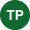# The Pythagorean Theorem: Delta Math Answer2 months ago

The Pythagorean theorem is one of the most famous and useful mathematical formulas in geometry. It relates the lengths of the sides of a right triangle, which is a triangle that has one angle of 90 degrees. The theorem states that the square of the hypotenuse (the longest side of the right triangle) equals the sum of the squares of the other two sides. In symbols, we can write:

a^2 + b^2 = c^2

A and b are the lengths of the legs (the shorter sides), and c is the hypotenuse length.

The Pythagorean theorem has many applications in real life, such as finding the distance between two points, calculating the height of a building, or determining the size of a TV screen. It also has many connections to other areas of mathematics, such as algebra, trigonometry, and calculus.

This blog post will explore some of the history, proofs, and examples of the Pythagorean theorem. We will also show you how to use Delta Math to practice and master this important concept.

History of the Pythagorean Theorem

The Pythagorean theorem is named after Pythagoras, a Greek philosopher and mathematician who lived in the 6th century BC. He is credited with being the first to prove the theorem, although there is some debate about whether he actually did it himself or learned it from someone else. Pythagoras also founded a religious and philosophical movement called Pythagoreanism, which believed that numbers and geometry had mystical and spiritual significance.

However, the Pythagorean theorem was not invented by Pythagoras or the Greeks. It was known by many ancient civilizations, such as the Babylonians, Egyptians, Indians, and Chinese, long before Pythagoras was born. Some of the oldest written records of the theorem date back to around 1800 BC in Mesopotamia. There is also evidence that the theorem was used in practical problems such as surveying and construction.

Proofs of the Pythagorean Theorem

There are many different ways to prove the Pythagorean theorem using various methods and tools. Some proofs are purely algebraic, while others use geometric shapes and figures. Some proofs are simple and elegant, while others are complex and ingenious. Some proofs are ancient and classic, while others are modern and original.

One of the most famous proofs of the Pythagorean theorem is attributed to Euclid, a Greek mathematician who lived in the 3rd century BC. He wrote a book called Elements, which is considered one of the most influential works in mathematics. His book presented a systematic and rigorous exposition of geometry based on axioms, definitions, propositions, and proofs. One of his propositions was the Pythagorean theorem, which he proved using a clever technique involving similar triangles.

Another well-known proof of the Pythagorean theorem is based on a visual representation called a proof without words. This proof uses a diagram that shows four copies of a right triangle arranged around a square. By comparing the areas of different parts of the diagram, we can see that the area of the square on the hypotenuse is equal to the sum of the areas of the squares on the legs.

Examples of the Pythagorean Theorem

Let's look at some examples to see how to use the Pythagorean theorem in practice.

Example 1: Find the length of the hypotenuse of a right triangle with legs of 3 cm and 4 cm.

Solution: Using the formula a^2 + b^2 = c^2, we can plug in the values of a and b and solve for c:

3^2 + 4^2 = c^2

9 + 16 = c^2

25 = c^2

c = sqrt(25)

c = 5

Therefore, the length of the hypotenuse is 5 cm.

Example 2: Find the length of one leg of a right triangle with a hypotenuse of 13 m and another leg of 12 m.

Solution: Using the same formula as before, we can plug in the values of c and b and solve for a:

a^2 + b^2 = c^2

a^2 + 12^2 = 13^2

a^2 + 144 = 169

a^2 = 169 - 144

a^2 = 25

a = sqrt(25)

a = 5

Therefore, the length of one leg is 5 m.

Example 3: Find the diagonal of a rectangle with width of 8 cm and length of 15 cm.

Solution: To find the diagonal of a rectangle, we can use the Pythagorean theorem by imagining that we cut the rectangle into two right triangles along the diagonal. Then, the diagonal becomes the hypotenuse of one of the triangles, and the width and length become the legs. Using the formula, we can plug in the values of a and b and solve for c:

a^2 + b^2 = c^2

8^2 + 15^2 = c^2

64 + 225 = c^2

289 = c^2

c = sqrt(289)

c = 17

Therefore, the diagonal of the rectangle is 17 cm.